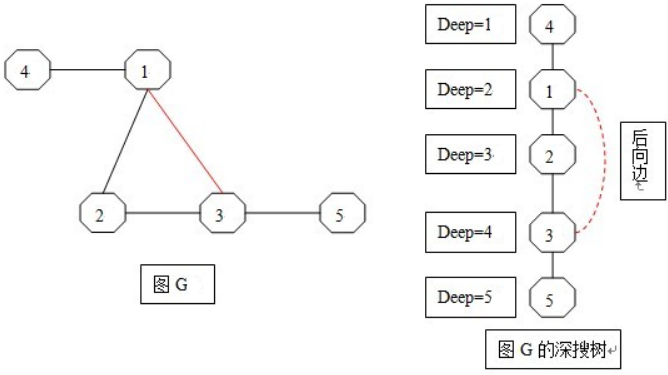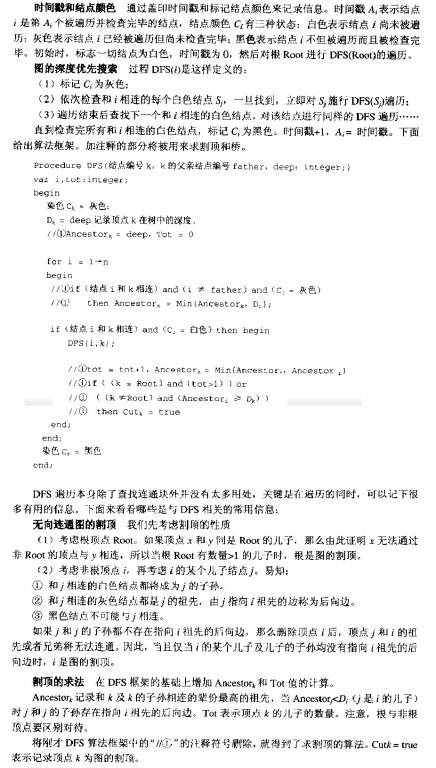# POJ 1523 - SPF

• POJ 1523 - SPF
• Time: 1000MS
• Memory: 10000K
• 难度: 中级
• 分类: 连通分量/割边/割点

## 解题思路

• 1、【割点】： 在一个无向连通图中，如果有一个顶点集合，删除这个顶点集合，以及这个集合中所有顶点相关联的边以后，原图变成多个连通块，就称这个点集为割点集合。当割点集合的顶点个数只有1个时，该顶点就是割点。
• 2、【连通分量】： 当删除某个割点后，原图会被划分为若干个互不连通的子图，这些子图就是该割点对应的连通分量。• （1）若k为深搜树的根Root，当且仅当k的儿子数（分支数）`>=2` 时k为割点；
• （2）若k为搜索树的中间结点（即k既不为根也不为叶），那么k必然有father和son，若 `AnceDeep[son] >= deep[k]`，则k必然为割点。

• 她的 `Ancestor[]` 就是我上文提及的 `AnceDeep[]`
• 她的 `D[]` 就是我上文提及的 `deep[]`
• 她的Tot就是我上文提及的son
• 她的deep就是时间戳，即搜索深度，我在程序中定义为depth，DFS时每入栈一次，`depth+1`，退栈一次 `depth-1`• 1、 用邻接表存储图。
• 2、注意输入输出格式，尤其是每组数据的“两个空格”和“一个空行”，以免PE。
• 3、测试数据并所给出的编号并不一定是从编号1开始的，因此一开始就应该开辟1000个（最大结点数）的存储空间，以免RE。

## AC 源码

``````//Memory Time
//280K   0MS

#include<iostream>
using namespace std;

const int size=1001;

struct Node
{
int id;                //结点编号
struct Node* next;
};

class solve
{
public:
solve():cases(0)
{
for(int i=0;i<size;i++)        //初始化邻接链表链头
ListTable_Head[i]=0;

DFS();
}
~solve()
{
delete[] *ListTable_Head;
}

int min(int a,int b) const{return a<b?a:b;}

bool Input(void);                            //返回0: 等待下一组输入。 返回1:程序结束
void Insert(int x,int y);                    //把无向边(x,y)插入邻接表

void DFS(void);                                //搜索割点及其对应的连通分量数
void DFS_SPF(int k,int father,int depth);    //搜索割点。k:当前结点。father:k的父亲结点。depth:搜索深度(时间戳)
void DFS_Subnet(int i);                        //i为从割点出发的分支,搜索并标记该从该分支出发所能到达的所有node
//（这些node就是一个连通分量）

void Empty(void);                            //清空邻接表(保留链头并使其指向NULL)
void Del(Node* p);                            //删除以结点p为链头的整条链(保留链头并使其指向NULL)

protected:

int cases;                        //案例数
Node* ListTable_Head[size];        //邻接链表链头

int Root;                        //深搜树的根(搜索起点)
int deep[size];                    //结点k的搜索深度deep[k]
int AnceDeep[size];                //结点k及其子孙辈分最高(深度最浅)的祖先的深度AnceDeep[k]
int color[size];                //结点k的颜色color[k]。0:未访问未检查；1:已访问未检查；2:已访问已检查
bool cut[size];                    //标记结点是否为割点
bool SPF;                        //标记网络是否出现割点

int Subnet_Num;                    //被割点的划分的连通分量的个数
bool vist[size];                //标记访问过的node
};

bool solve::Input(void)
{
int x,y;
for(int i=0;;i++)
{
cin>>x;
if(x==0 && i==0)        //程序结束
break;
else if(x==0 && i!=0)    //当前case的数据输入完毕
return true;

cin>>y;
Insert(x,y);
}
return false;
}

void solve::Insert(int x,int y)
{
if(!ListTable_Head[x])        //链头不存在，则创建
{
ListTable_Head[x]=new Node;
ListTable_Head[x]->next=0;
}
if(!ListTable_Head[y])
{
ListTable_Head[y]=new Node;
ListTable_Head[y]->next=0;
}

Node* px=ListTable_Head[x];
Node* py=ListTable_Head[y];
Node* tmp;

tmp=px->next;        //头插入法
px->next=new Node;
px->next->id=y;
px->next->next=tmp;

tmp=py->next;        //头插入法
py->next=new Node;
py->next->id=x;
py->next->next=tmp;

return;
}

void solve::DFS(void)
{
while(Input())
{
SPF=false;
memset(deep,0,sizeof(deep));
memset(AnceDeep,0,sizeof(AnceDeep));
memset(color,0,sizeof(color));
memset(cut,false,sizeof(cut));

/*搜索编号最小的node作为深搜树树的根*/
for(int k=1;k<size;k++)
if(ListTable_Head[k])
{
Root=k;
break;
}

/*寻找所有割点*/
DFS_SPF(Root,Root,1);

cout<<"Network #"<<++cases<<endl;
if(!SPF)
cout<<"  No SPF nodes"<<endl;
else
{
for(int i=Root;i<size;i++)  //小剪枝，根编号必定是最小的编号
if(cut[i])
{
Subnet_Num=0;
memset(vist,false,sizeof(vist));
vist[i]=true;

/*枚举割点i的所有分支，其中没有访问过的分支则对其逐一深搜*/
/*找出被割点i划分的所有连通分量*/
for(Node* p=ListTable_Head[i]->next;p;p=p->next)
{
if(!vist[p->id])        //分支p->id没有被访问
{                        //说明当前分支与前面搜索的连通分量 不连通
Subnet_Num++;        //则连通分量数+1
DFS_Subnet(p->id);    //搜索并标记该连通分量下的所有node
}
}
cout<<"  SPF node "<<i<<" leaves "<<Subnet_Num<<" subnets"<<endl;
}
}
cout<<endl;

Empty();
}
return;
}

void solve::DFS_SPF(int k,int father,int depth)
{
color[k]=1;                //染色，结点k已访问未检查
deep[k]=depth;            //记录k的搜索深度
AnceDeep[k]=depth;        //初始化,k最浅的祖先的深度就是k本身的深度
int son=0;                //k的儿子数

for(Node* p=ListTable_Head[k]->next;p;p=p->next)
{
int i=p->id;

if(color[i]==0)        //未访问未检查的node
{
son++;            //k的分支中，所有未访问未检查的node都是其儿子
DFS_SPF(i,k,depth+1);
AnceDeep[k]=min(AnceDeep[k],AnceDeep[i]);    //由于k和其儿子i必然同宗
}                                                //若在i中出现后向边使得i的祖先辈分更高（深度更浅）
//那么k的祖先辈分应该被更新

if(i!=father && color[i]==1)    //k的分支中，所有已访问未检查的node都是其祖先
AnceDeep[k]=min(AnceDeep[k],deep[i]);    //直接检查祖先辈分（深度）并更新

if((k==Root && son>1) ||            /*根结点的儿子数(分支数) >1时，则Root必定是割点*/
(k!=Root && AnceDeep[i]>=deep[k]))     /*k的儿子i的最大祖先的深度比k的深度要深，则删除k后i与k的father必然断开*/
{
cut[k]=true;
SPF=true;
}
}
color[k]=2;
return;
}

void solve::DFS_Subnet(int cp)
{
for(Node* p=ListTable_Head[cp]->next;p;p=p->next)
{
int i=p->id;
if(!vist[i])
{
vist[i]=true;
DFS_Subnet(i);
}
}
return;
}

void solve::Empty(void)
{
for(int i=1;i<size;i++)
{
if(ListTable_Head[i])
Del(ListTable_Head[i]);
ListTable_Head[i]=0;        //保留链表表头并初始化
}
return;
}

void solve::Del(Node* p)
{
if(p->next)
Del(p->next);
delete p;
return;
}

int main(void)
{
solve poj1523;
return 0;
}``````

## 相关资料

上一篇POJ 1010 - STAMPS
POJ 1010 - STAMPS Time: 1000MS Memory: 10000K 难度: 初级 分类: 搜索 问题描述题意比较难懂。大致如下： 第一行数字是邮票的面值，每一个数字就是一个不同的种类，哪怕面值相同。以0结束。 第
2011-03-07POJ 3009 - Curling 2.0
POJ 3009 - Curling 2.0 Time: 1000MS Memory: 65536K 难度: 初级 分类: DFS 问题描述哈哈，玩过“GBA口袋怪兽”的同学看到这题都应该非常容易理解了，“游戏也不过是这样写出来的” 哈
2011-02-28
目录